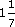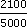Ratio, proportion and percentages

Start this free course now. Just create an account and sign in. Enrol and complete the course for a free statement of participation or digital badge if available.

Free course

# 3.2.1 Try some yourself

## Activity 20

Convert each of the following to percentages. Round off the percentages to whole numbers.

• (a)

• (i) 0.8

• (ii) 0.21

• (iii) 0.70

• (iv) 2.4

• (b)

• (i)• (ii)• (iii)• (iv)• (a)

• (i) 0.8 = 80%

• (ii) 0.21 = 21%

• (iii) 0.70 = 70%

• (iv) 2.4 = 240%

• (b)

• (i)= 0.5= 50%

• (ii)= 0.125=12.5%13%

• (iii)0.111111%

• (iv)1.143114%

## Activity 21

In the election in Example 11 the Average party gets 2100 votes. In the previous election they had 10%. Find the swing.

2100 out of 5000 is× 100% = 42%.

The swing is 42 − 10 = 32 percentage points.

MU120_4M3